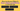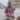# How to Access Object's Keys, Values, and Entries in JavaScript

Posted August 11, 2020Start discussion

You often need to look through the properties and values of plain JavaScript objects.

Here are the common lists to extract from an object:

• The keys of an object is the list of property names.
• The values of an object is the list of property values.
• The entries of an object is the list of pairs of property names and corresponding values.

Let’s consider the following JavaScript object:

``````const hero = {
name: 'Batman',
city: 'Gotham'
};``````

The keys of `hero` are `['name', 'city']`. The values are `['Batman', 'Gotham']`. And the entries are `[['name', 'Batman'], ['city', 'Gotham']]`.

Let’s see what utility functions provide JavaScript to extract the keys, values, and entries from an object.

## 1. Object.keys() returns keys

`Object.keys(object)` is a utility function that returns the list of keys of `object`.

Let’s use `Object.keys()` to get the keys of `hero` object:

``````const hero = {
name: 'Batman',
city: 'Gotham'
};

Object.keys(hero); // => ['name', 'city']``````

`Object.keys(hero)` returns the list `['name', 'city']`, which, as expected, are the keys of `hero` object.

### 1.1 Keys in practice: detect if object is empty

If you’d like to quickly check if an object is empty (has no own properties), then a good approach is to check whether the keys list is empty.

To check if the object is empty, all you need to do is verify the length property of the array returned by `Object.keys(object)`:

``const isObjectEmpty = Object.keys(object).length === 0;``

In the following example, `empty` has no properties, while `nonEmpty` object has one property:

``````const empty = {};
const nonEmpty = { a: 1 };

Object.keys(empty).length === 0;    // => true
Object.keys(nonEmpty).length === 0; // => false``````

`Object.keys(empty).length === 0` evaluates to `true`, which means that `empty` has no properties.

## 2. Object.values() returns values

`Object.values(object)` is the JavaScript utility function that returns the list of values of `object`.

Let’s use this function to get the values of `hero` object:

``````const hero = {
name: 'Batman',
city: 'Gotham'
};

Object.values(hero); // => ['Batman', 'Gotham']``````

`Object.values(hero)` returns the values of `hero`: `['Batman', 'Gotham']`.

### 2.1 Values in practice: calculate properties sum

`books` is an object that holds the prices of some books. The property key is the book name, while the value is the book price.

How would you determine the sum of all books from the object? By accessing the values of the object, and summing them.

Let’s see how to do that:

``````const books = {
'The Shining': 5.50,
'Harry Potter and the Goblet of Fire': 10.00,
'1984': 4.35
};

const prices = Object.values(books);

prices; // => [4.35, 5.5, 10]

const sum = prices.reduce((sum, price) => sum + price);

sum; // => 19.85``````

`Object.values(books)` returns the values of books object, which in this case is the `prices` list.

Then `prices.reduce(Math.sum)` summarizes the prices.

## 3. Object.entries() returns entries

Finally, `Object.entries(object)` is an useful function to access the entries of `object`.

Let’s extract the entries of `hero` object:

``````const hero = {
name: 'Batman',
city: 'Gotham'
};

Object.entries(hero); // => `[['name', 'Batman'], ['city', 'Gotham']]```````

`Object.entries(hero)` returns the entries of `hero`: `[['name', 'Batman'], ['city', 'Gotham']]`.

### 3.1 Entries in practice: find the property having 0 value

Again, let’s use the `books` object that holds the prices of some books. This time, due to a mistake, one of the books has been assigned with the price `0`.

Let’s find the book with the price `0` and log its name to console.

Using the object’s entries list fits well to solve this task:

``````const books = {
'The Shining': 5.50,
'Harry Potter and the Goblet of Fire': 10.00,
'1984': 0
};

for (const [book, price] of Object.entries(books)) {
if (price === 0) {
console.log(book);
}
}
// logs '1984'``````

`Object.entries(books)` returns a list of tuples: the book name and price. `const [book, price]` extracts in place from the tuple the book name and price.

Finally, inside the `for..of` cycle, you can check which book price is `0`, and log the name to console if that’s the case.

## 4. Summary

The keys, values, and entries are 3 common lists to extract from a JavaScript object for further processing.

JavaScript provides the necessary utility function to access these lists:

• The keys are returned by `Object.keys(object)`
• The values are returned by `Object.values(object)`
• And the entries are returned by `Object.entries(object)`

What other ways to access keys, values, and entries do you know?

## Quality posts into your inbox

I regularly publish posts containing:

• Important JavaScript concepts explained in simple words
• Overview of new JavaScript features
• How to use TypeScript and typing
• Software design and good coding practices